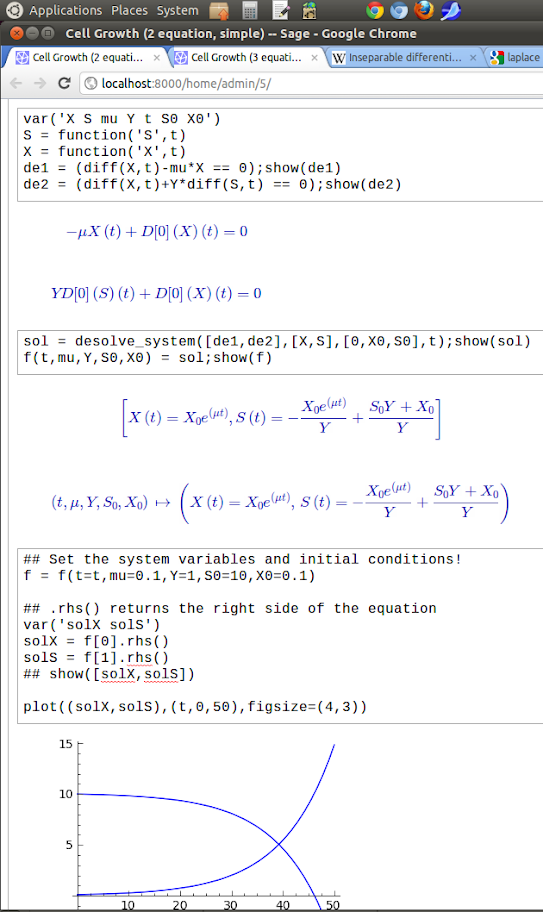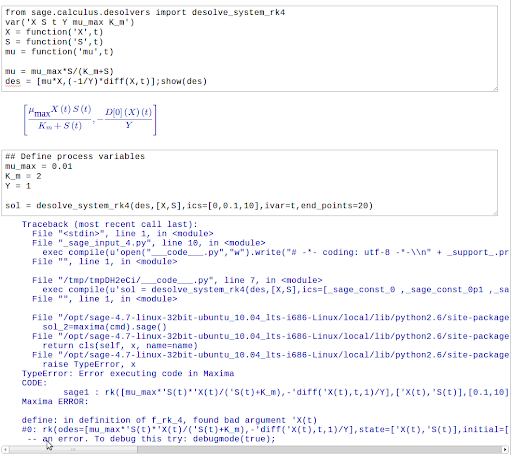Ask Your Question

# Solving a System of Differential Equations

I am attempting to solve and graph the solution to an initial value problem containing a system of differential equations. If I am remembering calculus correctly, its properties (nonlinear, ordinary, no explicit appearance of the independent variable time) classify it as a 'time-invariant autonomous system'.

I want to model a situation in which a microorganism in a bioreactor at some concentration 'X' is growing exponentially 'dX/dt = u*X' by consuming a substrate at some concentration 'S'.

Substrate consumption '-dS/dt' and microorganism growth rate 'dX/dt' are related by a constant representing the yield of biomass on substrate, Y. If I stop here and leave the specific growth rate 'u' a constant, I can evaluate this just fine with desolve_system():The problems start when I make 'u' (specific growth rate) a function of 'S' (substrate concentration):
u = u_max*S/(K_m+S).

I make the assumption that this must be evaluated numerically, and switch to desolve_system_rk4(). I get an "Error executing code in Maxima", which also states "in definition f_rk_4, found bad argument 'X(t)"Where am I going wrong? Can I use desolve_system_rk4() to evaluate this system, and I'm just making a syntax error?

Thank you for your patience - I just started using SAGE yesterday.

• Brandon
edit retag close merge delete

## 2 Answers

Sort by » oldest newest most voted

That function desolve_system_rk4 is very badly documented. I would suggest filing a bug report on the documentation.

That said, I think the answer to your question is that you have entered the DE in the wrong way. The list of expressions you pass to the solver need to be the right hand sides of equations you get after solving for $X'(t)$ and $S'(t)$ respectively. So instead of $\frac{-X'(t)}{Y}$ for the second expression, you want $$\frac{-0.01XS}{2+S}$$ . Then you don't need to define $X(t)$ and $S(t)$ as symbolic functions (which is I think where the error lies in your screenshot), they can remain just "variables". Here's an example:

sage: (X,S,t) = var('X,S,t')
sage: des = [0.01*X*S/(2+S), -0.01*X*S/(2+S)]
sage: desolve_system_rk4(des, [X,S], ics=[0,0.1,10], ivar=t, end_points=20)
[[0, 0.100000000000000, 10], [0.1, 0.100083368007, 9.99991663199],
[0.2, 0.100166805401, 9.9998331946], [0.3, 0.100250312238, 9.99974968776],
[0.4, 0.100333888577, 9.99966611142], [0.5, 0.100417534474, 9.99958246553],
...

more

## Comments

I don't know why the LaTeX expressions are giving me trouble in the answer... Maybe someone can point out the typesetting problem.

I don't know how to do this. Could you try re-defining f in the first place, without using mu as a variable but instead using the expression you've given?

Also, your question might be easier for someone else to answer if you copy/paste the function definitions (using the code format button to format it correctly here). Then people who want to help can just copy/paste to get their own versions to test with.

more

## Your Answer

Please start posting anonymously - your entry will be published after you log in or create a new account.

Add Answer

## Stats

Asked: 2011-07-20 20:44:36 +0200

Seen: 5,530 times

Last updated: Jul 23 '11